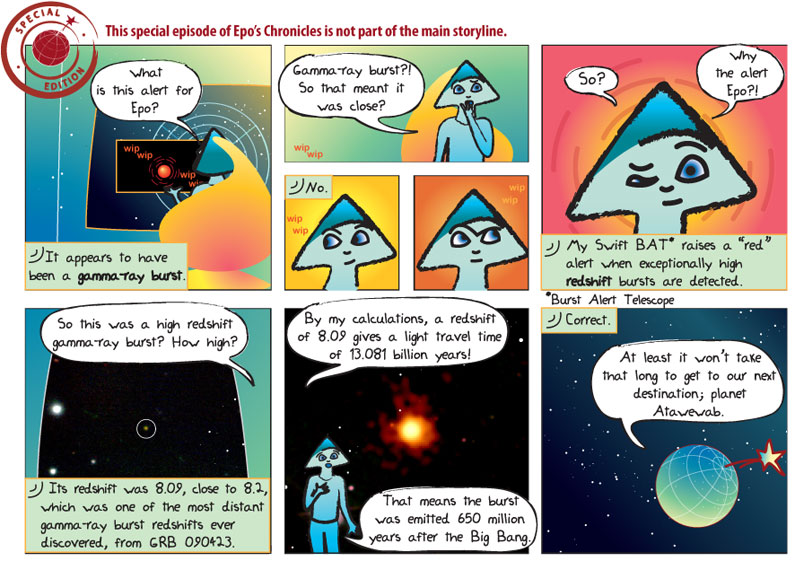## What does it mean?

Gamma-ray burst – a brief, but very energetic burst of the most powerful form of electromagnetic radiation.

Redshift (cosmological) – This is the name given to the apparent change in the wavelength of light due to the expansion of the universe. Scientists know what the spectrum of a galaxy should look like (based on the laboratory spectra of light emitted from known elements). In all but a few nearby galaxies, the familiar spectral lines are shifted to longer wavelengths than would be measured in a laboratory. Red light is at the long wavelength end of the visible spectrum, and blue light is at the short wavelength end, so shifts to longer wavelengths are called redshifts. The cosmological redshift is denoted by the letter z, and it is defined such that the universe has expanded by an amount 1+z over the time the light has traveled to us. So an object with redshift z=1 is seen when the universe was half its present size (it is twice as big as when the light was emitted), if z=2 the universe is three times bigger than when the light was emitted, if z=3 the universe is four times bigger, and so on.